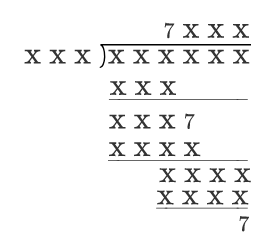# Feynman Long divisionThe above is a long division with most of the digits of any number hidden, except for the three 7's. Given that each of 0, 1, 2, ..., 9 was used at least once for the hidden digits, figure out all of the digits hiding and submit your answer as the value of the dividend (the 6-digit number being divided).

Details and Assumptions:

• Each $\mathrm X$ represents a single-digit integer.
• The leading (leftmost) digit of a number cannot be 0.
×